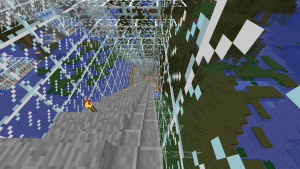Day: July 9, 2017

# Python in Minecraft 8 – stairs

To add stairs we need to know if the tunnel is descending or ascending to the east. We do this by creating a variable called yprev which stores the previous vertical position of the tunnel to the current position. We are creating the tunnel by increasing the value of x which means we are heading east. Hence if the current vertical position is more than the previous vertical position, the stairs are ascending to the east. If the current vertical position is less than the previous vertical position, the stairs are ascending to the west (descending to the east). If they are the same then we are not ascending or descending so no stairs are needed. At the end of the loop we need to store the current value of y in yprev so next time through the loop we will know the previous value. Check the full code to see where we initialise yprev before the loop.

```    # check if stairs are going up or down
if y > yprev:
# variation 0 = stairs ascending to the east
mc.setBlock(x,y,z-1,STAIRS_STONE.id,0)
if y < yprev:
# variation 1 = stairs ascending to the west
mc.setBlock(x-1,yprev,z-1,STAIRS_STONE.id,1)
yprev=y
```

We have decided to use stone stairs which are not predefined in Block.py in the modded mcpi. Hence we define it manually here.

```    STAIRS_STONE = block.Block(109)
```

Here is a screenshot showing the ascending stairs at the end of the tunnel.Here is the full code.

```import mcpi.minecraft as minecraft
import mcpi.block as block
mc=minecraft.Minecraft.create()
XMIN = -400 # x value at one end of tunnel.
XMAX = -200 # x value at other end of tunnel. Must be greater than XMIN
GROUND = 72 # y position of tunnel at each end
FLOOR = 10  # minimum value of y at bottom of tunnel
ZPOS = -244 # z position for full length of tunnel
TAIL = 10   # length of horizontal at each end of tunnel

# Define blocks currently missing from mcpi.block
STAIRS_STONE = block.Block(109)

# constants for tunnelvertical function
kx = (XMAX + XMIN) / 2
ky = GROUND + TAIL - (XMAX - XMIN) / 2

# tunnelvertical returns a vertical position of the tunnel
# for each value of the x position (eastposition)
def tunnelvertical(eastposition):
y = abs(eastposition - kx) + ky
if y < FLOOR:
return FLOOR
if y > GROUND:
return GROUND
return y

# set the z coordinate for the tunnel which doesn't change for the full length
z = ZPOS

# Initial loop to create a route made of solid glass with a stone base
for x in range(XMIN,XMAX+1):
y = tunnelvertical(x)
mc.setBlocks(x,y,z-2,x,y+6,z+2,block.GLASS)
mc.setBlocks(x,y,z-1,x,y,z+1,block.STONE)

# Initialise previous value of y so can determine if stairs going up or down
yprev = tunnelvertical(XMIN)
# Second loop to convert solid glass into a tunnel
for x in range(XMIN+1,XMAX):
y = tunnelvertical(x)
# replace centre glass with air to make it a tunnel
mc.setBlocks(x,y+1,z-1,x,y+5,z+1,block.AIR)
# place a torch every 4 positions to light the tunnel
# variation 5 = torch facing up
if x % 4 == 0:
mc.setBlock(x,y+1,z+1,block.TORCH.id,5)
# check if stairs are going up or down
if y > yprev:
# variation 0 = stairs ascending to the east
mc.setBlock(x,y,z-1,STAIRS_STONE.id,0)
if y < yprev:
# variation 1 = stairs ascending to the west
mc.setBlock(x-1,yprev,z-1,STAIRS_STONE.id,1)
yprev=y
```
Categories: CoderDojo